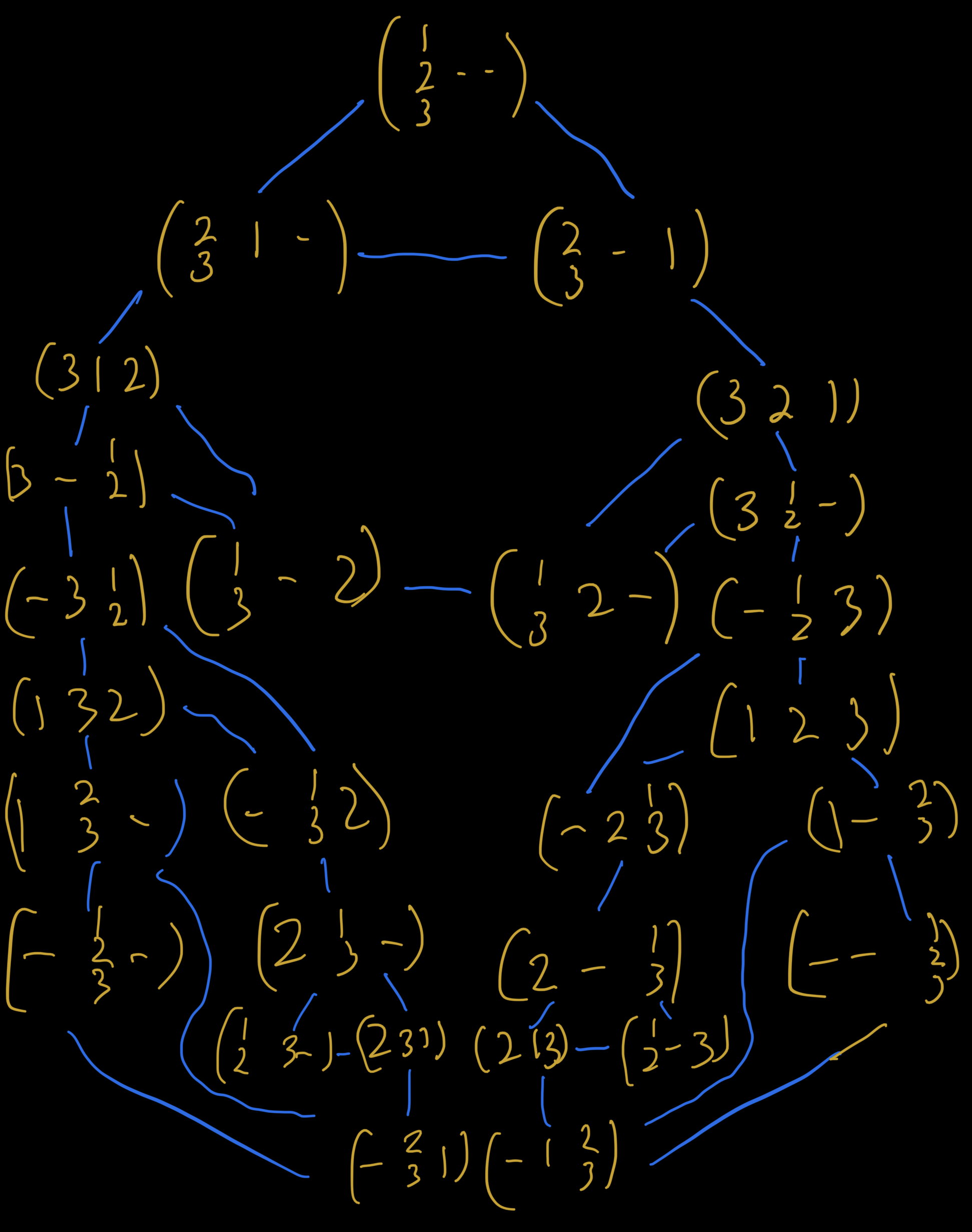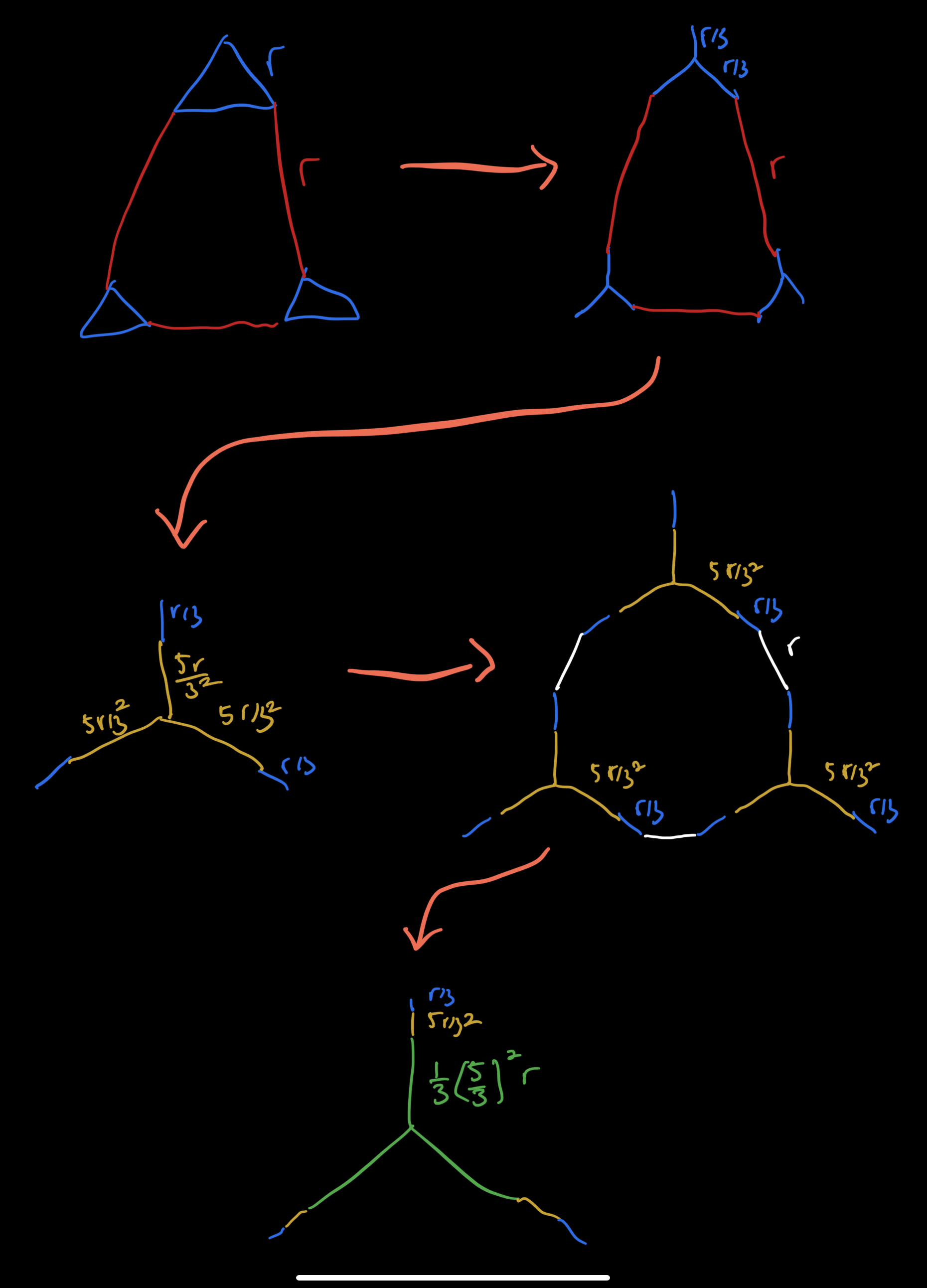Question: it’s now one year into quarantine and you’re well and truly out of ideas for fun on a Saturday night. To avoid falling asleep, you set up three pegs with three rings, each wider than the next, stacked widest to narrowest on the first peg. You want to move this stack to either of the other two pegs, moving one disk at a time, such that you never put a wider disk on top of a narrower disk. You know this is hard, but you have nothing but time. Suppose that instead of analyzing the situation, you simply move the disks at random, choosing uniformly from among the available valid moves at each step. How long should you expect to be at this exciting new amusement?

## Solution

Liberated from the burden of strategy, we are free to be our true selves and write down the state space for the arrangements of the tower, of which there are $27$ valid game states: ($\left(123,-,-\right)$ three ways, $\left(13, 2, -\right)$ six ways, $\left(12, 3, -\right)$ six ways, $\left(23, 1, -\right)$ six ways, and $\left(1, 2, 3\right)$ six ways). Each state can only reach $3$ other states unless it’s a solved state in which case it has just $2$ neighbors. writing them all out, we get the interesting topology of a Sierpinski gasket:The problem is a random walk from the top of this graph down to either of the corners.

The symmetry in the graph suggests that it can be massively simplified, reminiscent of many a resistor puzzle. At first I looked for ways to join similar edges, reducing the topology to a line, in hopes to map onto gambler’s ruin, like I did in the thanksgiving puzzle, but I couldn’t get that to go.

I then looked for a recursion, after all the graph has three identical subgraphs (ignoring the peg states). In fact, the expected time to arrive at an endstate from a node does have a nice relationship with the expected time to arrive at an endstate from one of its neighbors, though it isn’t recursive.

At node $x$, the expected time for me to get to node $y$ is $T(x\rightarrow y).$ From node $x$, I can step randomly to any of my $d(x)$ neighbors. So, $T(x\rightarrow y)$ can be written in terms of its neighbors expectation times. Writing down the time it takes to get from node $x$ to node $y,$ we get

$T(x \rightarrow y) = \frac{1}{d(x)} \sum_n \left(1 + T(n \rightarrow y)\right),$

where the sum is over all the neighbors $n$ of $x.$ This is a harmonic function (nearest neighbors average), just like the voltage at a node in a resistor circuit.

At a node $x$ in a circuit, the current from a neighbor $n$ is equal to $(v(n) - v(x))/r_{xn}$ or, with unit resistors, $(v(n) - v(x)),$ and the total current flowing out of a node is $\sum_n (v(n) - v(x)),$ which has to be zero. So, $v(x) \times d(x) = \sum_n v(n)$ leads to $v(x) = \frac{1}{d(x)} \sum_n v(n).$ Inspecting, we can substract the voltage at node $y$ from both sides to get

$v(x) - v(y) = 1 + \frac{1}{d(x)} \sum_n (v(n) - v(y)).$

$f(x,y) = T(x \rightarrow y) - (v(x) - v(y))$ is also harmonic and zero when $x$ is $y$ ($v(x)$ and $v(x)$ have the same voltage, $T(x \rightarrow x)$ takes no time), so, $f(x,y)$ is uniformly zero, and $T(x\rightarrow y)$ and $(v(x) - v(y))$ are the same function.

This suggests there’s a mapping from $T(x, y)$ onto an equivalent resistor circuit wherein $T(x\rightarrow y)$ amounts to a reduction through the symmetries of series and parallel combinations of edges.

If we can find a situation where we know the current from node $x$ to node $y,$ then we can use Ohm’s law

$v(x) - v(y) = I_{xy} R_{xy}$

to find $T(x \rightarrow y).$ We can do this by superposition, e.g.

• first inject some amount of current into the graph while node $x$ is held to zero voltage, all nodes $z$ will realize some voltage $v(z)$ relative to $x$
• next, set up the same scenario at $y$, i.e., hold node $y$ to zero voltage and collect current from it, then reverse all currents (so that we’re injecting at node $y$)
• overlay the two grids so that current flows only from node $x$ to node $y.$ All the other current flows cancel.

But how much current should we inject? Rearranging the harmonic relation connecting neighboring voltages, we get

\begin{align} d(j) &= d(j)v(j) - \sum_n v(n) \\ &= \sum_n \left(v(j) - v(n)\right)) \end{align}

But the sum of $(v(j) - v(n))$ over all neighbors $n$ is just the current emerging from the node $j$ when the set of voltages is maintained. So, $d(j) = i_\text{inject at$j$}.$

So, if we inject $d(j)$ to every node $j$ then, in the overlaid circuits,

$2T(x\rightarrow y) = \sum_j d(j) = \text{twice number of edges}$

will be injected at node $x$ and $\text{twice number of edges}$ will be extracted at node $y,$ sp

$\boxed{T(x \rightarrow y) = \text{number of edges}\times R_{xy}}.$

At the end, we just have to find the resistance between the top and bottom corner of the state space. And, since we can go to either corner, this means that

\begin{align} T(\text{pole 1} \rightarrow \text{pole 2 or 3}) &= 1/2 \times T(\text{pole 1} \rightarrow \text{pole 2}) \\ &= 1/2 \times T(\text{pole 1} \rightarrow \text{pole 3}). \end{align}

In the first three steps below, we reduce the upper third of the game board diagram to a three-way junction. The next step pieces three of those together (to form the full three peg + three disk board) and the last step reduces that to its own three way junction.The resistance between pegs $1$ and $3,$ $R_{13},$ is then the series sum of resistance along the path. The pattern is already pretty clear from the three series elements:

\begin{align} R_{13} &= \frac{r}{3}\sum\limits_0^{n-1} \left(\frac{5}{3}\right)^n \\ &= \frac{r}{3} \dfrac{1 - \left(\frac{5}{3}\right)^n}{1-\frac{5}{3}} \\ &= \frac{r}{2}\left[\left(\frac{5}{3}\right)^n - 1\right]. \end{align}

As we noted at the top, there are $27$ game states, $24$ of which have degree $3$ and $3$ of which have degree $2,$ and in general, twice the number of edges in the Sierpinski circuit is $\left(3^n - 3\right)\times 3 + 3\times 2.$

Putting this altogether, the expected time to travel from a stack on peg $1$ to a stack on one of the other pegs is

$\overbrace{\frac{1}{2}\times T(1\rightarrow 3)}^{v(x)-v(y)} = \overbrace{\frac{1}{2}\times\frac{1}{2}\left[\left(\frac{5}{3}\right)^n - 1\right]}^{R_{xy}}\overbrace{\times\left(\left(3^n - 3\right)\times 3 + 3\times 2\right)}^{I_{xy}}$

which is $32/3\approx 10.7$ for two disks, $637/9\approx 70.8$ for three disks, $10880/27\approx 403.0$ for four disks, and $174361/81\approx 2152.6$ for five disks.